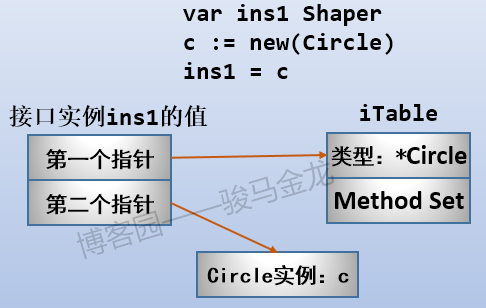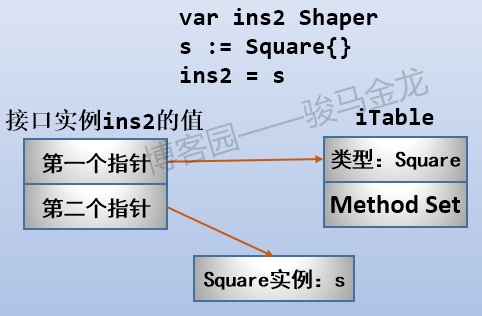# Go基础系列：Go接口

By | 2018年11月2日

## 接口用法简介

``````type Namer interface {
my_method1()
my_method2(para)
my_method3(para) return_type
...
}``````

``````package main

import (
"fmt"
)

// Shaper 接口类型
type Shaper interface {
Area() float64
}

// Circle struct类型
type Circle struct {
}

// Circle类型实现Shaper中的方法Area()
func (c *Circle) Area() float64 {
}

// Square struct类型
type Square struct {
length float64
}

// Square类型实现Shaper中的方法Area()
func (s *Square) Area() float64 {
return s.length * s.length
}

func main() {
// Circle类型的指针类型实例
c := new(Circle)

// Square类型的值类型实例
s := Square{3.2}

// Sharpe接口实例ins1，它只能是值类型的
var ins1 Shaper
// 将Circle实例c赋值给接口实例ins1
// 那么ins1中就保存了实例c
ins1 = c
fmt.Println(ins1)

// 使用类型推断将Square实例s赋值给接口实例
ins2 := s
fmt.Println(ins2)
}``````

``````&{2.5}
{3.2}``````

``````ins1 = c
ins2 := s``````

``````fmt.Println(ins1.Area())   // 输出19.625
fmt.Println(ins2.Area())   // 输出10.24``````

## 接口实例中存的是什么

``````println(ins1)
println(c)``````

``````(0x4ceb00,0xc042068058)
0xc042068058``````

ins中的第一个是指针是什么？它所指向的是一个内部表结构iTable，这个Table中包含两部分：第一部分是实例c的类型信息，也就是`*Circle`，第二部分是这个类型(Circle)的方法集，也就是Circle类型的所有方法(此示例中Circle只定义了一个方法Area())。## 方法集(Method Set)规则

``````实例的类型       receiver
--------------------------------------
值类型:T       (T Type)
指针类型:*T    (T Type)或(T *Type)``````

• 值类型的实例的Method Set只由值类型的receiver`(T Type)`组成
• 指针类型的实例的Method Set由值类型和指针类型的receiver共同组成，即`(T Type)``(T *Type)`

``````receiver        实例的类型
---------------------------
(T Type)        T 或 *T
(T *Type)       *T``````

• 如果某类型实现接口的方法的receiver是`(T *Type)`类型的，那么只有指针类型的实例`*T`才算是实现了这个接口
• 如果某类型实现接口的方法的receiver是`(T Type)`类型的，那么值类型的实例`T`和指针类型的实例`*T`都算实现了这个接口

``````package main

import "fmt"

// Shaper 接口类型
type Shaper interface {
Area() float64
}

// Circle struct类型
type Circle struct {
}

// Circle类型实现Shaper中的方法Area()
func (c *Circle) Area() float64 {
}

func main() {
// 声明2个接口实例
var ins1, ins2 Shaper

// Circle的指针类型实例
c1 := new(Circle)
ins1 = c1
fmt.Println(ins1.Area())

// Circle的值类型实例
c2 := Circle{3.0}
// 下面的将报错
ins2 = c2
fmt.Println(ins2.Area())
}``````

``````cannot use c2 (type Circle) as type Shaper
in assignment:
Circle does not implement Shaper (Area method has

``ins2 = &c2``

``````type Square struct{
length float64
}

func (s Square) Area() float64{
return s.length * s.length
}

func main() {
var ins3,ins4 Shaper

// 值类型的Square实例s1
s1 := Square{3.0}
ins3 = s1
fmt.Println(ins3.Area())

// 指针类型的Square实例s2
s2 := new(Square)
s2.length=4.0
ins4 = s2
fmt.Println(ins4.Area())
}``````

``````c2 = Circle{3.2}
ins2 := c2
fmt.Println(ins2.Area())  // 正常执行````````````package main

import "fmt"

type myint int

func (m *myint) add() myint {
return *m + 1
}
func main() {
}``````

``````abc\abc.go:11:22: cannot call pointer method on myint(3)
abc\abc.go:11:22: cannot take the address of myint(3)``````

## 接口类型作为参数

``````package main

import (
"fmt"
)

// Shaper 接口类型
type Shaper interface {
Area() float64
}

// Circle struct类型
type Circle struct {
}

// Circle类型实现Shaper中的方法Area()
func (c *Circle) Area() float64 {
}

func main() {
// Circle的指针类型实例
c1 := new(Circle)
myArea(c1)
}

func myArea(n Shaper) {
fmt.Println(n.Area())
}``````

``````\$ go doc fmt Println
func Println(a ...interface{}) (n int, err error)``````

## 接口类型的嵌套

``````type ReadWrite interface {
Write(b Buffer) bool
}
type Lock interface {
Lock()
Unlock()
}
type File interface {